# Recursion Chapter 5 Outline Induction Linear recursion Example

• Slides: 50Recursion Chapter 5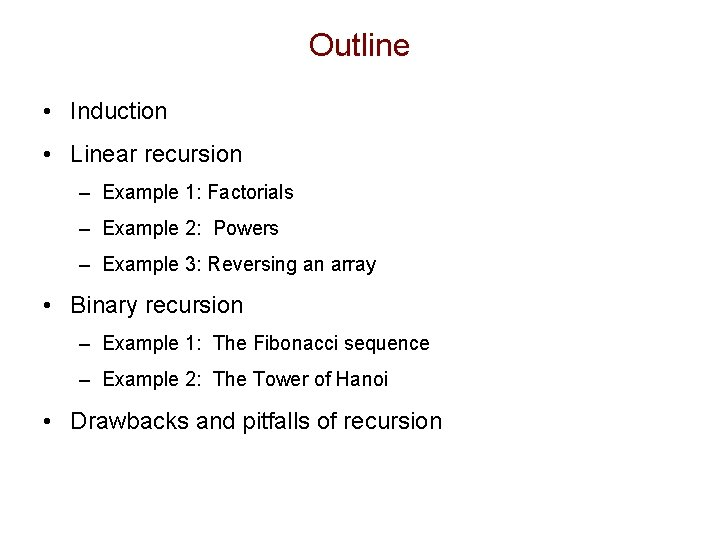Outline • Induction • Linear recursion – Example 1: Factorials – Example 2: Powers – Example 3: Reversing an array • Binary recursion – Example 1: The Fibonacci sequence – Example 2: The Tower of Hanoi • Drawbacks and pitfalls of recursion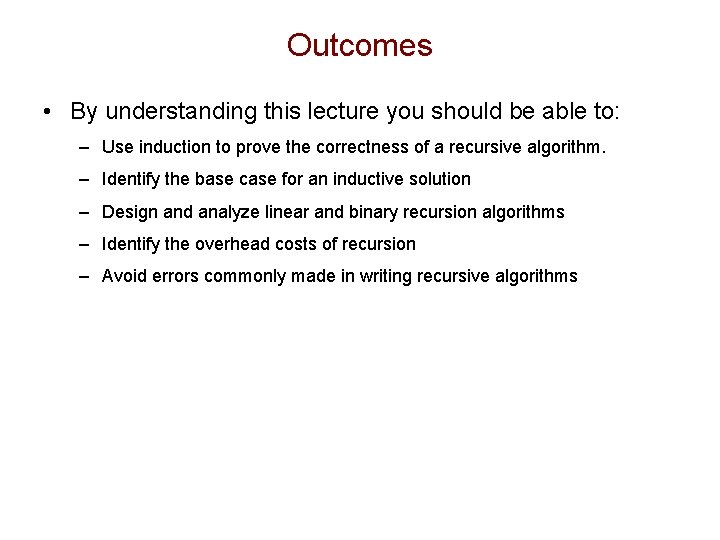Outcomes • By understanding this lecture you should be able to: – Use induction to prove the correctness of a recursive algorithm. – Identify the base case for an inductive solution – Design and analyze linear and binary recursion algorithms – Identify the overhead costs of recursion – Avoid errors commonly made in writing recursive algorithmsOutline • Induction • Linear recursion – Example 1: Factorials – Example 2: Powers – Example 3: Reversing an array • Binary recursion – Example 1: The Fibonacci sequence – Example 2: The Tower of Hanoi • Drawbacks and pitfalls of recursionDivide and Conquer • When faced with a difficult problem, a classic technique is to break it down into smaller parts that can be solved more easily. • Recursion uses induction to do this.History of Induction • Implicit use of induction goes back at least to Euclid’s proof that the number of primes is infinite (c. 300 BC). • The first explicit formulation of the principle is due to Pascal (1665). Euclid of Alexandria, "The Father of Geometry" c. 300 BC Blaise Pascal, 1623 - 1662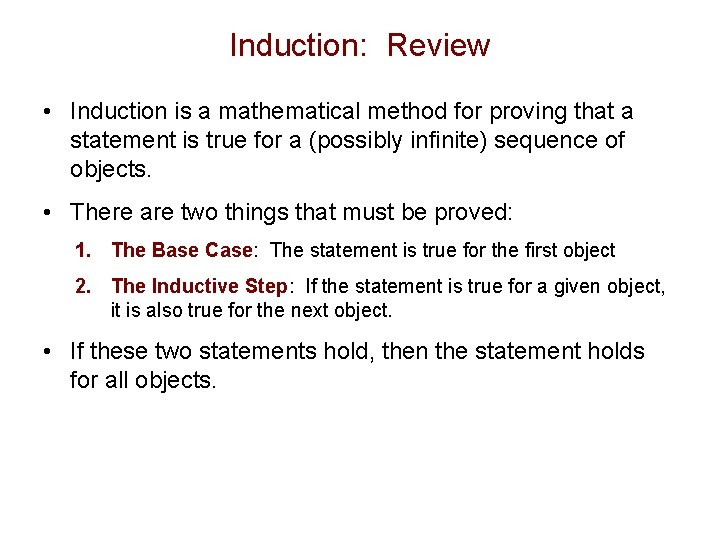Induction: Review • Induction is a mathematical method for proving that a statement is true for a (possibly infinite) sequence of objects. • There are two things that must be proved: 1. The Base Case: The statement is true for the first object 2. The Inductive Step: If the statement is true for a given object, it is also true for the next object. • If these two statements hold, then the statement holds for all objects.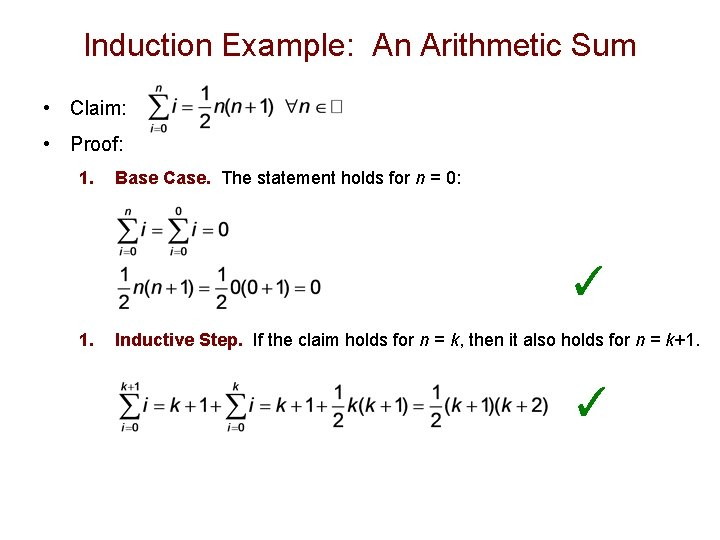Induction Example: An Arithmetic Sum • Claim: • Proof: 1. Base Case. The statement holds for n = 0: ✓ 1. Inductive Step. If the claim holds for n = k, then it also holds for n = k+1. ✓Recursive Divide and Conquer • You are given a problem input that is too big to solve directly. • You imagine, – “Suppose I had a friend who could give me the answer to the same problem with slightly smaller input. ” – “Then I could easily solve the larger problem. ” • In recursion this “friend” will actually be another instance (clone) of yourself. Tai (left) and Snuppy (right): the first puppy clone.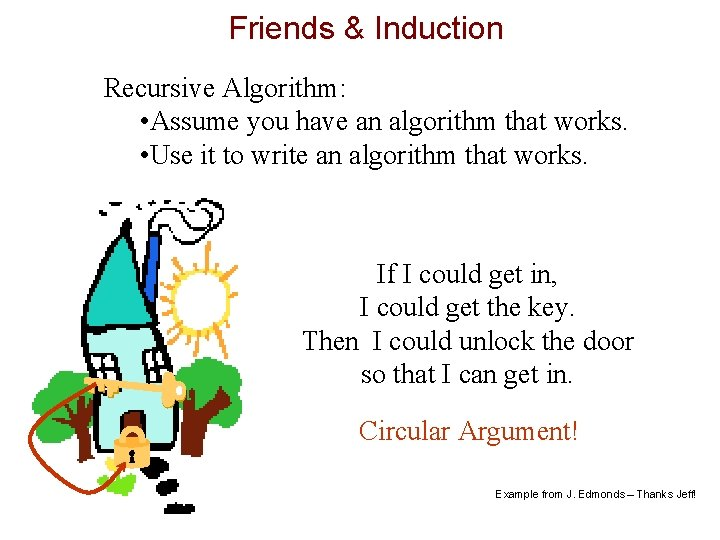Friends & Induction Recursive Algorithm: • Assume you have an algorithm that works. • Use it to write an algorithm that works. If I could get in, I could get the key. Then I could unlock the door so that I can get in. Circular Argument! Example from J. Edmonds – Thanks Jeff!Friends & Induction Recursive Algorithm: • Assume you have an algorithm that works. • Use it to write an algorithm that works. To get into my house I must get the key from a smaller houseFriends & Induction Recursive Algorithm: • Assume you have an algorithm that works. • Use it to write an algorithm that works. The “base case” Use brute force to get into the smallest house.Outline • Induction • Linear recursion – Example 1: Factorials – Example 2: Powers – Example 3: Reversing an array • Binary recursion – Example 1: The Fibonacci sequence – Example 2: The Tower of Hanoi • Drawbacks and pitfalls of recursion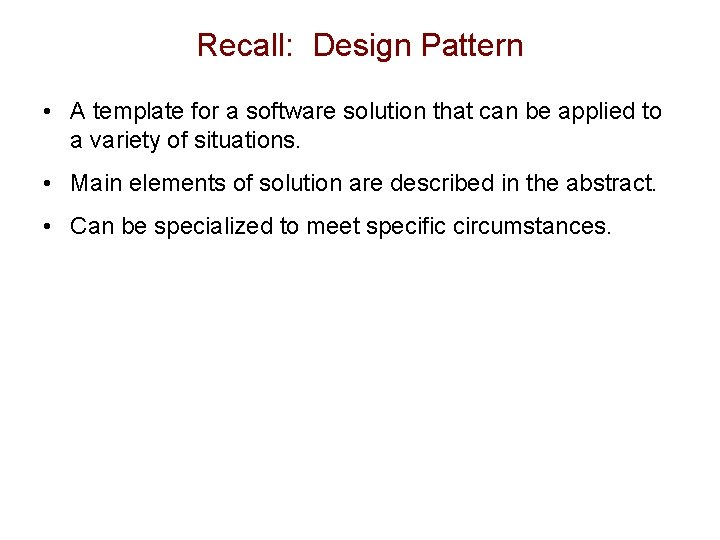Recall: Design Pattern • A template for a software solution that can be applied to a variety of situations. • Main elements of solution are described in the abstract. • Can be specialized to meet specific circumstances.Linear Recursion Design Pattern • Test for base cases – Begin by testing for a set of base cases (there should be at least one). – Every possible chain of recursive calls must eventually reach a base case, and the handling of each base case should not use recursion. • Recurse once – Perform a single recursive call. (This recursive step may involve a test that decides which of several possible recursive calls to make, but it should ultimately choose to make just one of these calls each time we perform this step. ) – Define each possible recursive call so that it makes progress towards a base case.Example 1 • The factorial function: – n! = 1· 2· 3· ··· · (n-1)· n • Recursive definition: • As a Java method: // recursive factorial function public static int recursive. Factorial(int n) { if (n == 0) return 1; // base case else return n * recursive. Factorial(n- 1); // recursive case }Tracing Recursion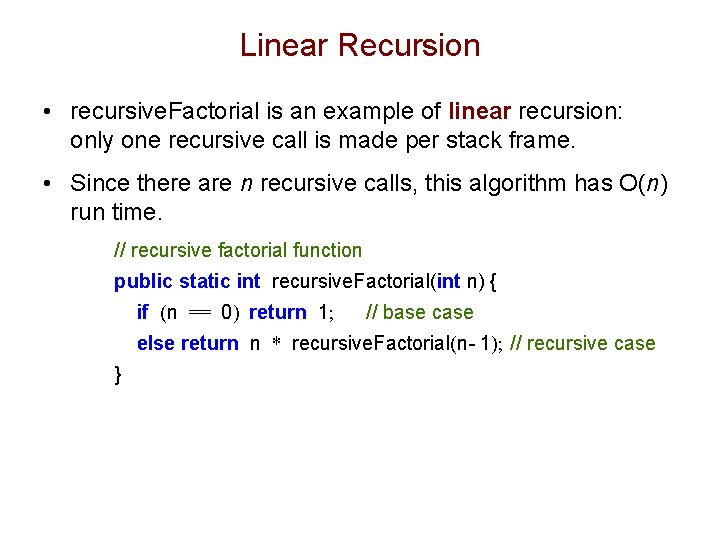Linear Recursion • recursive. Factorial is an example of linear recursion: only one recursive call is made per stack frame. • Since there are n recursive calls, this algorithm has O(n) run time. // recursive factorial function public static int recursive. Factorial(int n) { if (n == 0) return 1; // base case else return n * recursive. Factorial(n- 1); // recursive case }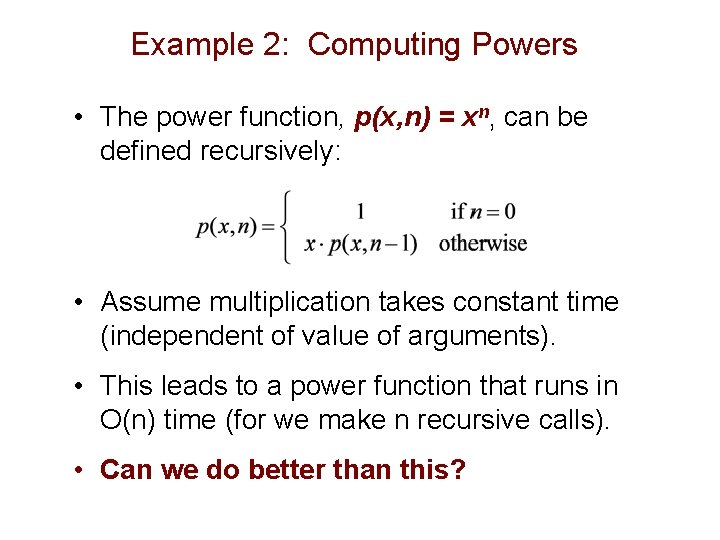Example 2: Computing Powers • The power function, p(x, n) = xn, can be defined recursively: • Assume multiplication takes constant time (independent of value of arguments). • This leads to a power function that runs in O(n) time (for we make n recursive calls). • Can we do better than this?Recursive Squaring • We can derive a more efficient linearly recursive algorithm by using repeated squaring: • For example, 24 = 2(4/2)2 = (22)2 = 42 = 16 25 = 21+(4/2)2 = 2(22)2 = 2(42) = 32 26 = 2(6/ 2)2 = (26/2)2 = (23)2 = 82 = 64 27 = 21+(6/2)2 = 2(23)2 = 2(82) = 128.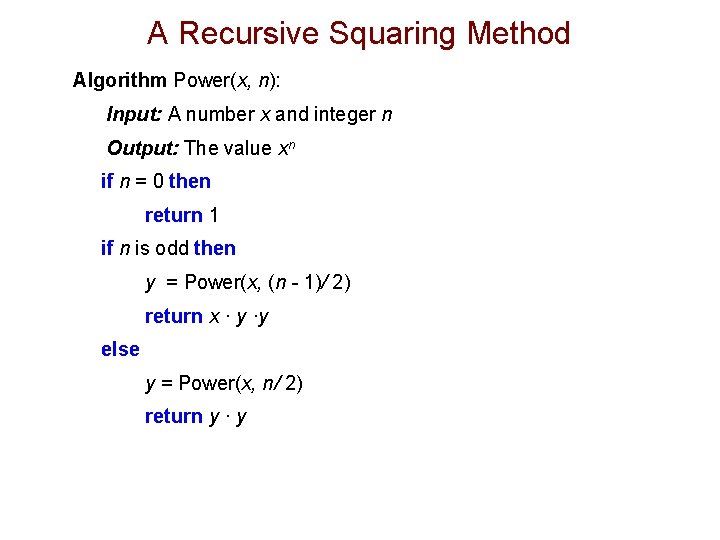A Recursive Squaring Method Algorithm Power(x, n): Input: A number x and integer n Output: The value xn if n = 0 then return 1 if n is odd then y = Power(x, (n - 1)/ 2) return x · y ·y else y = Power(x, n/ 2) return y · yAnalyzing the Recursive Squaring Method Algorithm Power(x, n): Input: A number x and integer n = 0 Output: The value xn if n = 0 then return 1 if n is odd then y = Power(x, (n - 1)/ 2) return x · y ·y else y = Power(x, n/ 2) return y · y Although there are 2 statements that recursively call Power, only one is executed per stack frame. Each time we make a recursive call we halve the value of n (roughly). Thus we make a total of log n recursive calls. That is, this method runs in O(log n) time.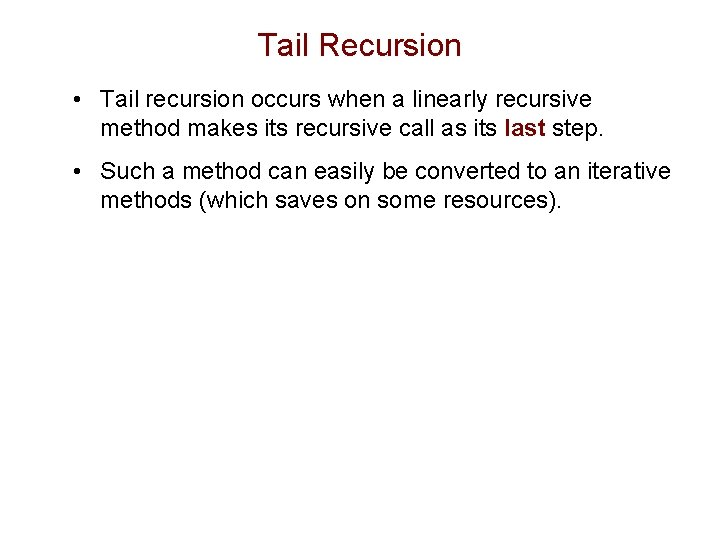Tail Recursion • Tail recursion occurs when a linearly recursive method makes its recursive call as its last step. • Such a method can easily be converted to an iterative methods (which saves on some resources).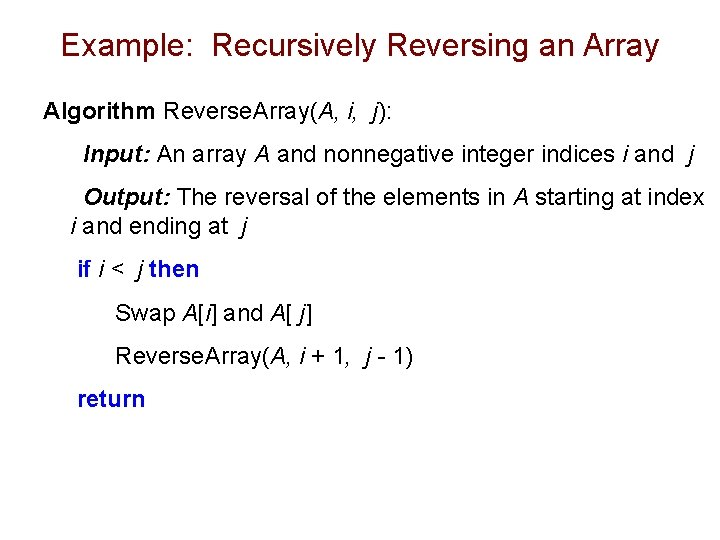Example: Recursively Reversing an Array Algorithm Reverse. Array(A, i, j): Input: An array A and nonnegative integer indices i and j Output: The reversal of the elements in A starting at index i and ending at j if i < j then Swap A[i] and A[ j] Reverse. Array(A, i + 1, j - 1) return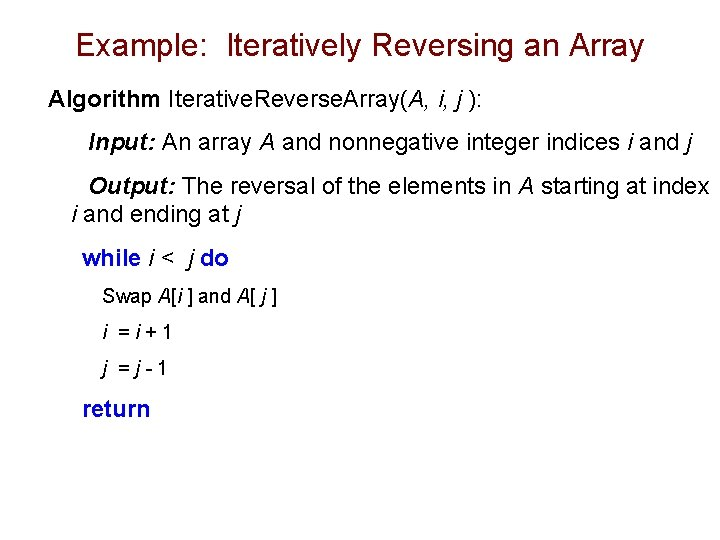Example: Iteratively Reversing an Array Algorithm Iterative. Reverse. Array(A, i, j ): Input: An array A and nonnegative integer indices i and j Output: The reversal of the elements in A starting at index i and ending at j while i < j do Swap A[i ] and A[ j ] i =i+1 j =j-1 returnDefining Arguments for Recursion • Solving a problem recursively sometimes requires passing additional parameters. • Reverse. Array is a good example: although we might initially think of passing only the array A as a parameter at the top level, lower levels need to know where in the array they are operating. • Thus the recursive interface is Reverse. Array(A, i, j). • We then invoke the method at the highest level with the message Reverse. Array(A, 1, n).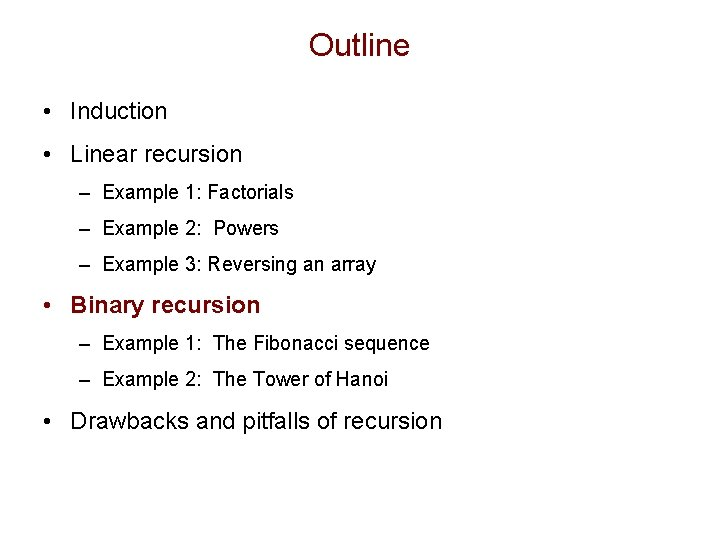Outline • Induction • Linear recursion – Example 1: Factorials – Example 2: Powers – Example 3: Reversing an array • Binary recursion – Example 1: The Fibonacci sequence – Example 2: The Tower of Hanoi • Drawbacks and pitfalls of recursion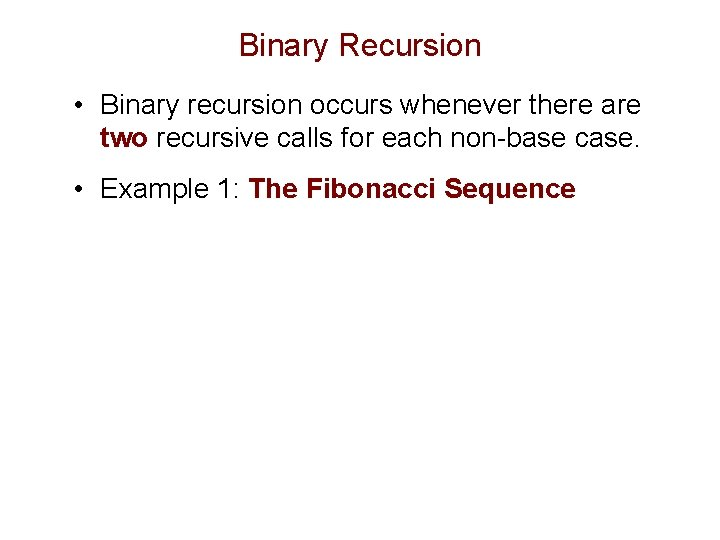Binary Recursion • Binary recursion occurs whenever there are two recursive calls for each non-base case. • Example 1: The Fibonacci SequenceThe Fibonacci Sequence • Fibonacci numbers are defined recursively: F 0 = 0 F 1 = 1 Fi = Fi-1 + Fi-2 for i > 1. (The “Golden Ratio”) Fibonacci (c. 1170 - c. 1250) (aka Leonardo of Pisa)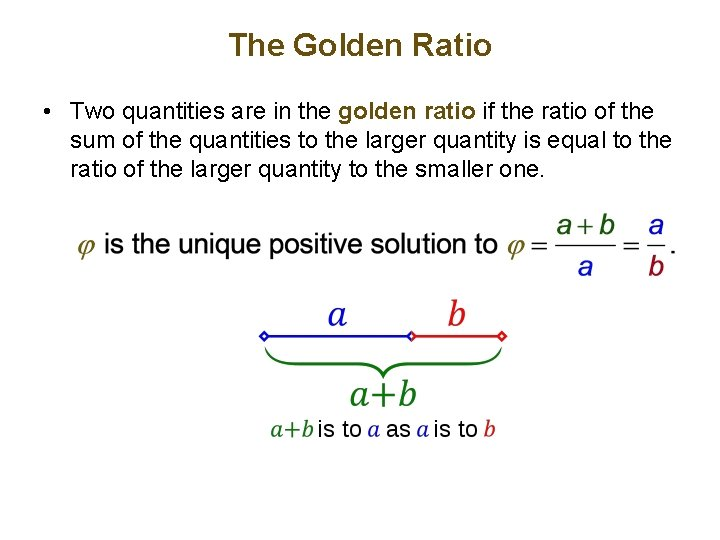The Golden Ratio • Two quantities are in the golden ratio if the ratio of the sum of the quantities to the larger quantity is equal to the ratio of the larger quantity to the smaller one.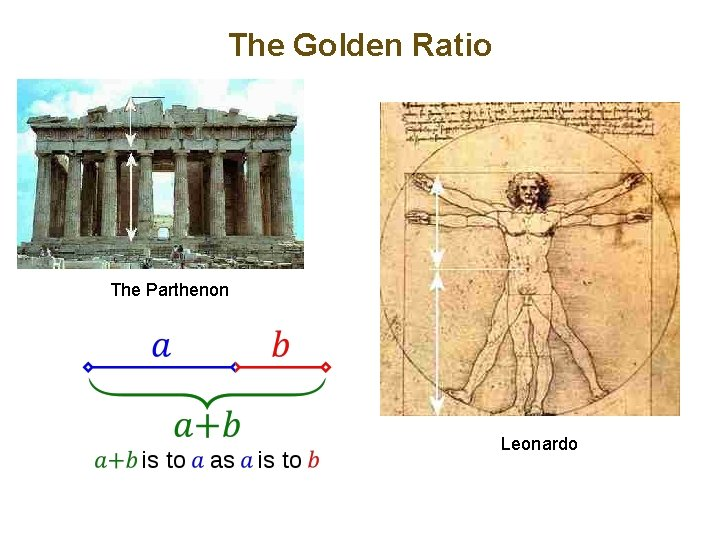The Golden Ratio The Parthenon LeonardoComputing Fibonacci Numbers F 0 = 0 F 1 = 1 Fi = Fi-1 + Fi-2 for i > 1. • A recursive algorithm (first attempt): Algorithm Binary. Fib(k): Input: Positive integer k Output: The kth Fibonacci number Fk if k < 2 then return k else return Binary. Fib(k - 1) + Binary. Fib(k - 2)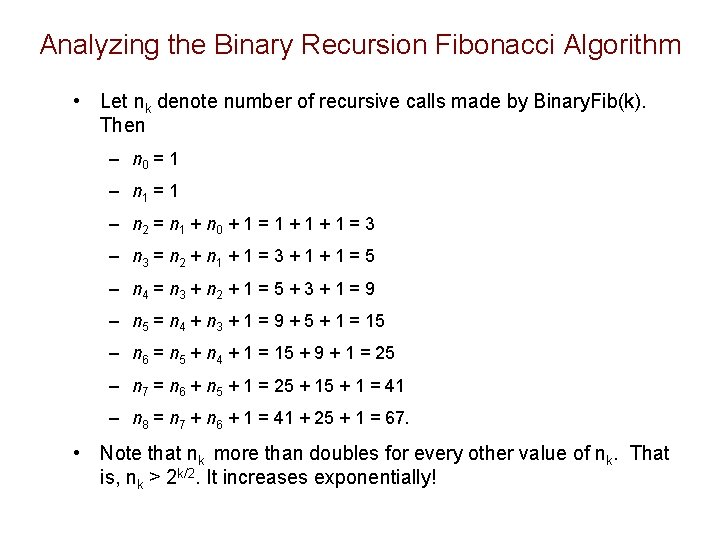Analyzing the Binary Recursion Fibonacci Algorithm • Let nk denote number of recursive calls made by Binary. Fib(k). Then – n 0 = 1 – n 1 = 1 – n 2 = n 1 + n 0 + 1 = 1 + 1 = 3 – n 3 = n 2 + n 1 + 1 = 3 + 1 = 5 – n 4 = n 3 + n 2 + 1 = 5 + 3 + 1 = 9 – n 5 = n 4 + n 3 + 1 = 9 + 5 + 1 = 15 – n 6 = n 5 + n 4 + 1 = 15 + 9 + 1 = 25 – n 7 = n 6 + n 5 + 1 = 25 + 1 = 41 – n 8 = n 7 + n 6 + 1 = 41 + 25 + 1 = 67. • Note that nk more than doubles for every other value of nk. That is, nk > 2 k/2. It increases exponentially!A Better Fibonacci Algorithm • Use linear recursion instead: Algorithm Linear. Fibonacci(k): Input: A positive integer k Output: Pair of Fibonacci numbers (Fk, Fk-1) if k = 1 then return (k, 0) Linear. Fibonacci(k): Fk, Fk-1 else (i, j) = Linear. Fibonacci(k - 1) return (i +j, i) • Runs in O(k) time. Linear. Fibonacci(k-1): Fk-1, Fk-2Binary Recursion • Second Example: The Tower of HanoiExample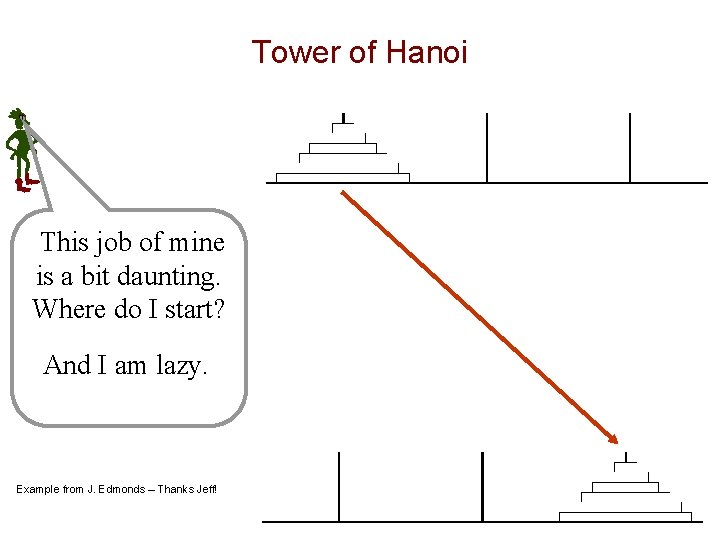Tower of Hanoi This job of mine is a bit daunting. Where do I start? And I am lazy. Example from J. Edmonds – Thanks Jeff!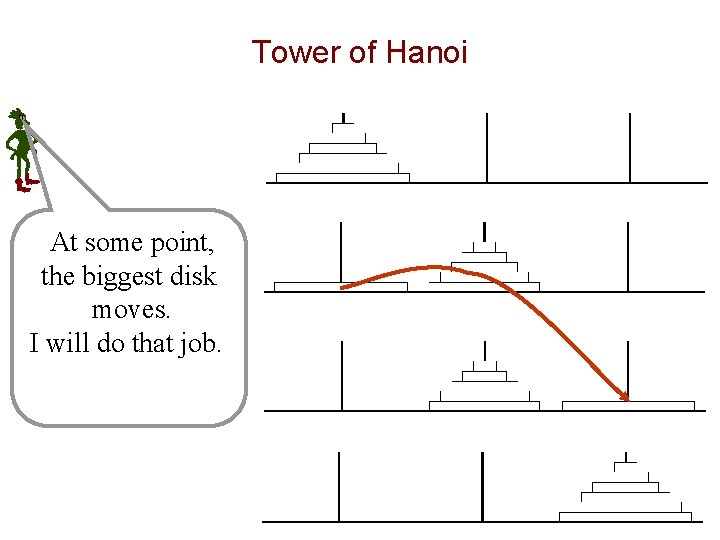Tower of Hanoi At some point, the biggest disk moves. I will do that job.Tower of Hanoi To do this, the other disks must be in the middle.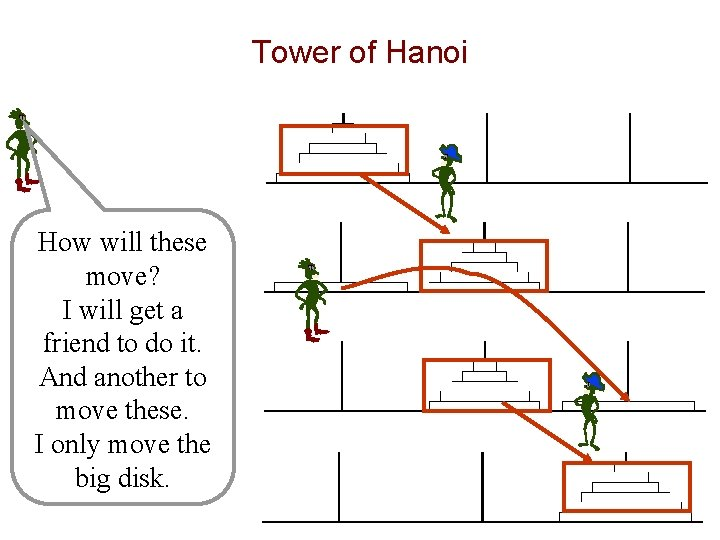Tower of Hanoi How will these move? I will get a friend to do it. And another to move these. I only move the big disk.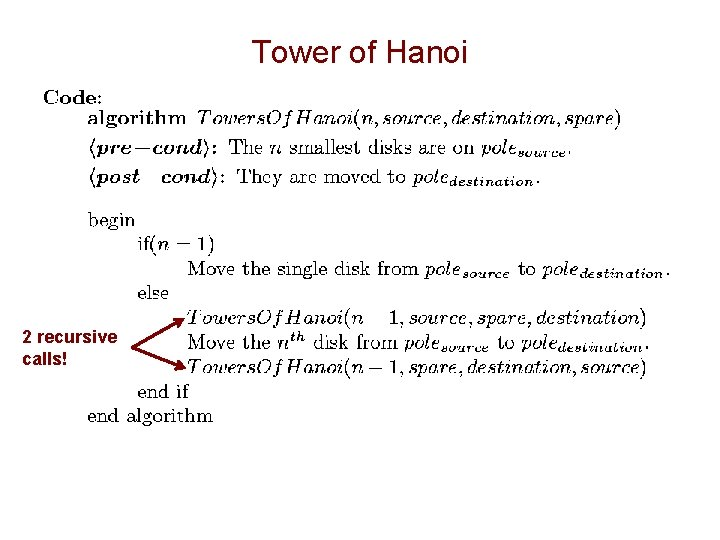Tower of Hanoi 2 recursive calls!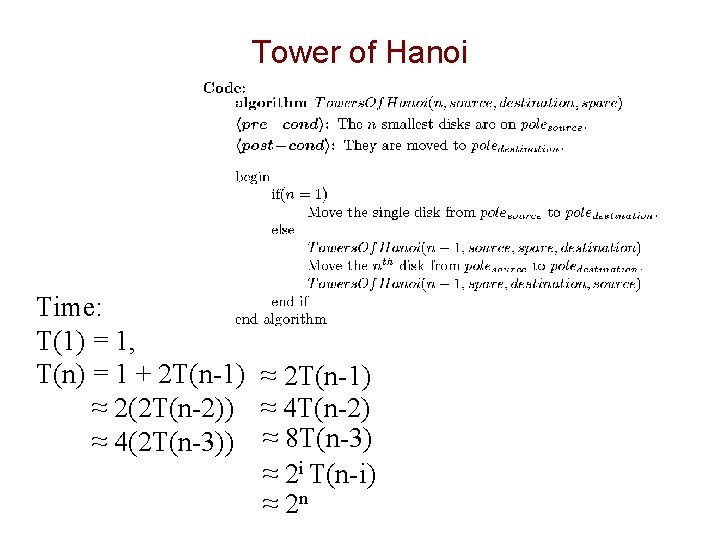Tower of Hanoi Time: T(1) = 1, T(n) = 1 + 2 T(n-1) ≈ 2(2 T(n-2)) ≈ 4 T(n-2) ≈ 4(2 T(n-3)) ≈ 8 T(n-3) ≈ 2 i T(n-i) ≈ 2 n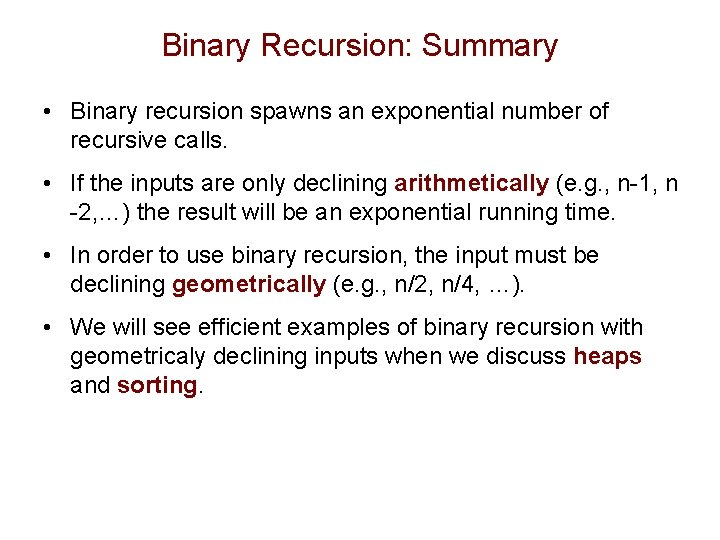Binary Recursion: Summary • Binary recursion spawns an exponential number of recursive calls. • If the inputs are only declining arithmetically (e. g. , n-1, n -2, …) the result will be an exponential running time. • In order to use binary recursion, the input must be declining geometrically (e. g. , n/2, n/4, …). • We will see efficient examples of binary recursion with geometricaly declining inputs when we discuss heaps and sorting.Outline • Induction • Linear recursion – Example 1: Factorials – Example 2: Powers – Example 3: Reversing an array • Binary recursion – Example 1: The Fibonacci sequence – Example 2: The Tower of Hanoi • Drawbacks and pitfalls of recursion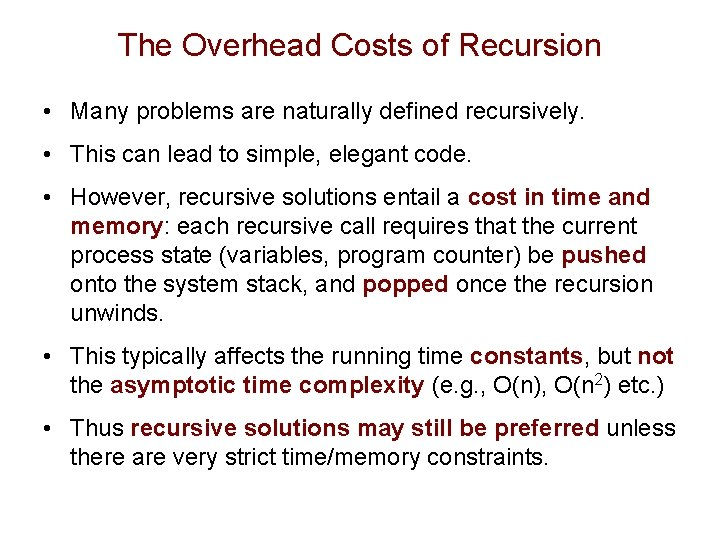The Overhead Costs of Recursion • Many problems are naturally defined recursively. • This can lead to simple, elegant code. • However, recursive solutions entail a cost in time and memory: each recursive call requires that the current process state (variables, program counter) be pushed onto the system stack, and popped once the recursion unwinds. • This typically affects the running time constants, but not the asymptotic time complexity (e. g. , O(n), O(n 2) etc. ) • Thus recursive solutions may still be preferred unless there are very strict time/memory constraints.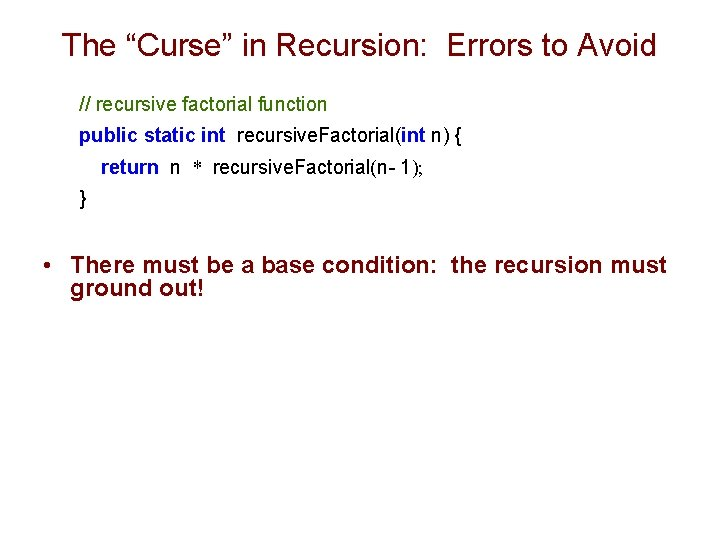The “Curse” in Recursion: Errors to Avoid // recursive factorial function public static int recursive. Factorial(int n) { return n * recursive. Factorial(n- 1); } • There must be a base condition: the recursion must ground out!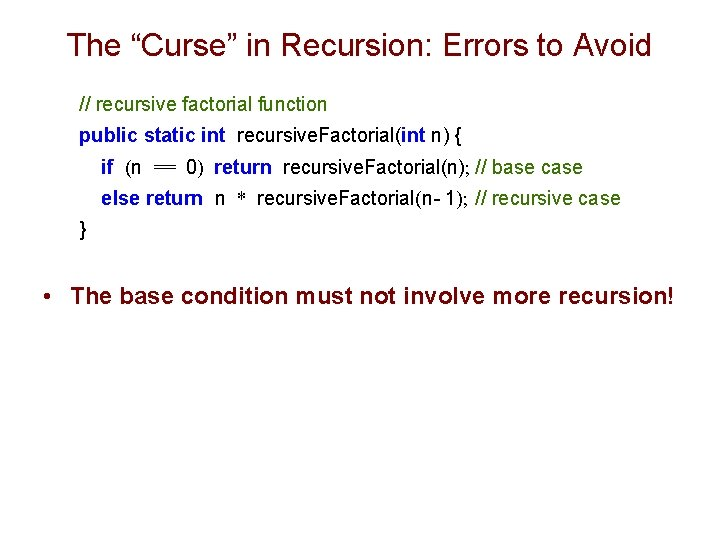The “Curse” in Recursion: Errors to Avoid // recursive factorial function public static int recursive. Factorial(int n) { if (n == 0) return recursive. Factorial(n); // base case else return n * recursive. Factorial(n- 1); // recursive case } • The base condition must not involve more recursion!The “Curse” in Recursion: Errors to Avoid // recursive factorial function public static int recursive. Factorial(int n) { if (n == 0) return 1; // base case else return (n – 1) * recursive. Factorial(n); case // recursive } • The input must be converging toward the base condition!Outline • Induction • Linear recursion – Example 1: Factorials – Example 2: Powers – Example 3: Reversing an array • Binary recursion – Example 1: The Fibonacci sequence – Example 2: The Tower of Hanoi • Drawbacks and pitfalls of recursionOutcomes • By understanding this lecture you should be able to: – Use induction to prove the correctness of a recursive algorithm. – Identify the base case for an inductive solution – Design and analyze linear and binary recursion algorithms – Identify the overhead costs of recursion – Avoid errors commonly made in writing recursive algorithms